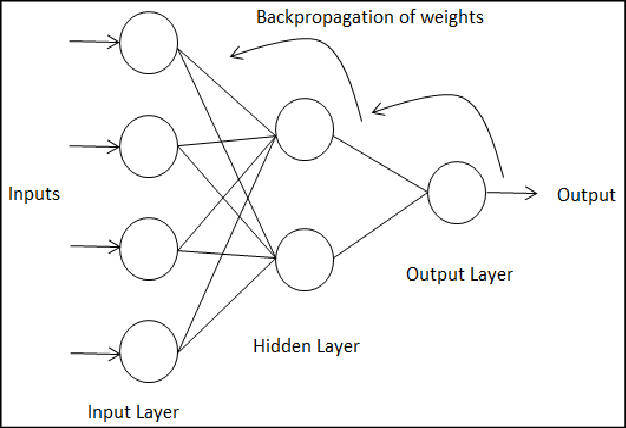/  Deep Learning Interview questions and answers   /  How do Neural Networks update weights and Biases during Back Propagation?## How do Neural Networks update weights and Biases during Back Propagation?

Ans. To reduce the error by changing the values of weights and biases.

calculate the rate of change of error w.r.t change in weight.Since we are propagating backwards, first thing we need to do is, calculate the change in total errors w.r.t the output O1 and O2.Now, we will propagate further backwards and calculate the change in output O1 w.r.t to its total net input.Let’s see now how much does the total net input of O1 changes w.r.t  W5?Step – 3: Putting all the values together and calculating the updated weight value

Now, let’s put all the values together:Let’s calculate the updated value of W5:This is an example to know how to update the weights and biases in Neural Network. This process continues until we get minimized error.